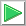# Signal Classification in the frequency domain.

 Tip In some cases it is possible to apply the Fourier Transform to the data before using it for training or validation. If data exhibits some periodic behavior, it is even possible to eliminate some frequency components without losing information. Neural Lab includes the function realfft to transform the data to the frequency domain. This function stores the real and imaginary part of the transformed data sequentially and works only when the length of the data is a power of two. See Wintempla > Signal Procesing > Fourier Transform for more information about the Fourier Transform.

 Problem 1 Create a New Project called SigFreq (You must select: Multi-layer Network and Classification in the New Project Dialog) to classify signals in the frequency domain. Edit the BuiltTrainSet.lab file to build an appropriate training set for the classification of the two waves: the sine and the saw tooth (see figure). Use 1000 training cases and 64 inputs. The input training set must include 500 sine waves and 500 saw tooth waves. Each training case has a wave with a random phase.Solution 1 After editing the file,Run click the button to execute the code. If you do not have any errors, the training set will be generated and displayed on the variable list and the file list. Click on the file to see its contents.

 SigFreq\BuildTrainSet.lab int numCases = 1000/2; int numInputs = 64; double deltaInput = 6.28/numInputs; int i; int j; double phase = 0.0; //________________________________ Class 1: sine Matrix inputSine; inputSine.Create(numCases, numInputs); Matrix targetSine; targetSine.Create(numCases, 2); for(i=0; iProblem 2 Edit the BuilValidSet.lab file to build an appropriate validation set for problem1. Use 400 validation cases and 64 inputs. The validation set input must include 150 sine waves, and 250 saw tooth waves. Each validation case has a wave with random phase and 10 % of noise. Be sure to transform the data to the frequency domain.

 Solution 2 After editing the file,Run click the button to execute the code. You will be asked to save the file, set the file name to BuildValidSet.lab. If you do not have any errors, the validation set will be generated and displayed on the variable list and the file list. Use the scroll bar at the right to visualize each validation case.

 SigFreq\BuildValidSet.lab int numInputs = 64; double deltaInput = 6.28/numInputs; int i; int j; double phase = 0.0; //________________________________ Class 1: sine int numCasesSine = 150; Matrix inputSine; inputSine.Create(numCasesSine, numInputs); Matrix targetSine; targetSine.Create(numCasesSine, 2); for(i=0; i

 Problem 3 Edit the Train.lab file to design and train an ANN for the classification of the sine wave and the saw tooth.

 Solution 3 After editing the file,Run click the button to execute the code. You will be asked to save the file, set the file name to Train.lab. If you do not have any errors, the ANN will be trained when the code execution stops. Double click the network in the variable list to see the network. Then, select the trainSetInput.csv file and, the trainSetTarget.csv file. You will be able to see the network behavior in real time by moving the scrollbar at the right.

 SigFreq\Train.lab //_________________________ Network Setup LayerNet net; net.Create(64, 2, 0, 2); // 2 Outputs means 2 Classes //________________________ Load the training set Matrix trainSetInput; trainSetInput.Load(); Matrix trainSetTarget; trainSetTarget.Load(); int i = 0; //____________________________ Input Scaling //net.AutoSetInScaler(trainSetInput); for(i = 0; i<64; i++) {      net.SetInScaler(i, -32.0, 32.0); } //____________________________ Output Scaling net.SetOutScaler(0, 0.0, 1.0); // Output values are from 0 to 1 net.SetOutScaler(1, 0.0, 1.0); // Output values are from 0 to 1 //________________________ Set the training set net.SetTrainSet(trainSetInput, trainSetTarget, false); //________________________ Train net.TrainSimAnneal(100, 100, 15, 0.01, false, 4, 1.0e-12); net.TrainLevenMar(2000,1.0e-12); //_____________________________ Save the trained network net.Save();Problem 4 Edit the CheckTraining.lab file to check the training: (a) Compute the confusion matrix using the training set. (b) Plot the error for each network output. (c) Save the confusion matrix as a vector image (trainConf.emf).

 Solution 4 (a) After editing the file,Run click the button to execute the code. If you do not have any errors, the number of errors will be displayed in the variable list. Click the variable called trainConf to view the confusion matrix for the training set.

 SigFreq\CheckTraining.lab //_________________________________________ Load the Training Set Matrix trainSetInput; trainSetInput.Load(); Matrix trainSetTarget; trainSetTarget.Load(); //_________________________________________ Load the ANN LayerNet net; net.Load(); //_________________________________________ Run Matrix output = net.Run(trainSetInput); //_________________________________________ Compute the Confusion Matrix Matrix trainConf = ConfusionMatrix(output, trainSetTarget, 0.5); trainConf.Save(); //_________________________________________ Compute the Number of Errors int numErrors = toint(trainConf.GetSum()) - toint(trainConf.GetDiagonalSum());Problem 5 Edit the Validation.lab file to perform the validation of the ANN. (a) Compute the confusion matrix using the validation set. (b) Plot the error for each network output. (c) Save the confusion matrix as a vector image (validConf.emf).

 Solution 5 (a) After editing the file,Run click the button to execute the code. You will be asked to save the file, set the file name to Validation.lab. If you do not have any errors, the number of errors will be displayed in the variable list. Click the variable called validConf to view the confusion matrix for the validation set.

 SigFreq\Validation.lab //_________________________________________ Load the validation set Matrix validSetInput; validSetInput.Load(); Matrix validSetTarget; validSetTarget.Load(); //_________________________________________ Load the ANN LayerNet net; net.Load(); //_________________________________________ Run Matrix output = net.Run(validSetInput); //_________________________________________ Compute the confusion matrix Matrix validConf = ConfusionMatrix(output, validSetTarget, 0.5); validConf.Save(); //_________________________________________ Compute the Number of Errors int numErrors = toint(validConf.GetSum()) - toint(validConf.GetDiagonalSum());Problem 6 Generate a report in Microsoft Word. Write some conclusions in the report focusing on the problems that were faced during the simulation and how these problems were or could be solved.